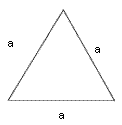Home > Geometry calculators > Area of a Equilateral Triangle calculator

Area -
Area - Equilateral TriangleFormula : Perimeter (P) = 3 aArea (A) = sqrt(3)/4 a^2

11. I know that for a equilateral triangles = . From this find out of the equilateral triangles.
Decimal Place =

 SolutionExampleArea FormulaAll Problems